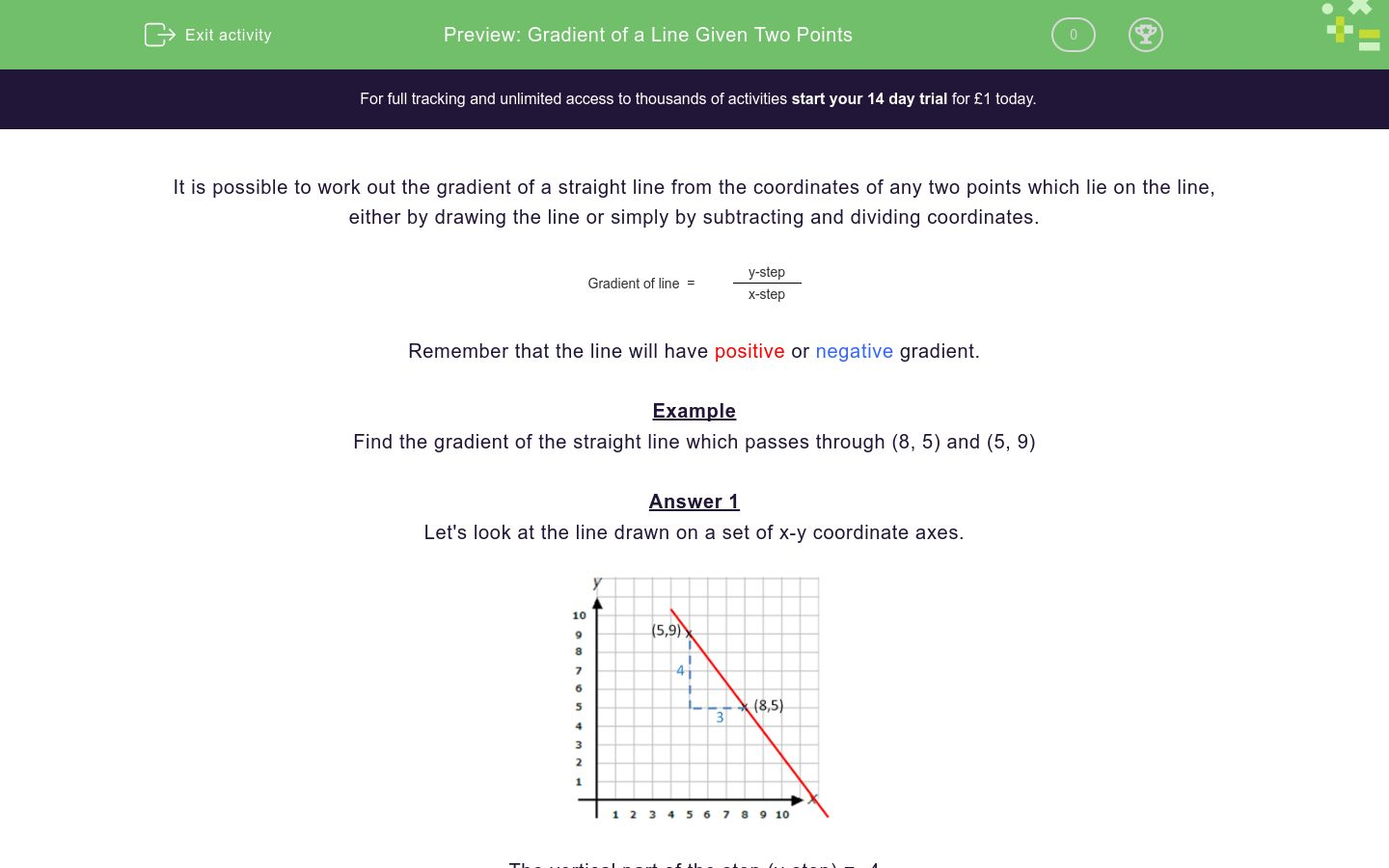# Gradient of a Line Given Two Points

In this worksheet, students calculate the gradient of a line which passes through two given points.Key stage:  KS 4

Curriculum topic:  Algebra

Curriculum subtopic:  Use y = mx + c to Identify Parallel and Perpendicular Lines

Difficulty level:### QUESTION 1 of 10

It is possible to work out the gradient of a straight line from the coordinates of any two points which lie on the line, either by drawing the line or simply by subtracting and dividing coordinates.

 Gradient of line  = y-step x-step

Remember that the line will have positive or negative gradient.

Example

Find the gradient of the straight line which passes through (8, 5) and (5, 9)

Let's look at the line drawn on a set of x-y coordinate axes.The vertical part of the step (y-step) = -4

The horizontal part of the step (x-step) = 3

 Gradient of line  = y-step = -4 x-step 3

It is possible to work out the gradient WITHOUT drawing the line.

Look at the coordinates of the two points (8, 5) and (59).

We can get the y-step by subtracting the y-coordinates.

y-step = 5 - 9 = -4

In the same order, we get the x-step by subtracting the x-coordinates:

x-step = 8 - 5 = 3

 Gradient of line  = y-step = -4 x-step 3

NB  We could have worked out 9 - 5 = 4 and 5 - 8 = -3 but 4 ÷ -3 = -4 ÷ 3

Find the gradient of the straight line which passes through:

(1, 5) and (5, 9)

(If it is not a whole number, write it as a fraction in the form a/b)

Find the gradient of the straight line which passes through:

(4, 12) and (5, 10)

(If it is not a whole number, write it as a fraction in the form a/b)

Find the gradient of the straight line which passes through:

(4, 2) and (5, 10)

(If it is not a whole number, write it as a fraction in the form a/b)

Find the gradient of the straight line which passes through:

(4, 2) and (5, -1)

(If it is not a whole number, write it as a fraction in the form a/b)

Find the gradient of the straight line which passes through:

(3, 8) and (5, 5)

(If it is not a whole number, write it as a fraction in the form a/b)

Find the gradient of the straight line which passes through:

(1, 2) and (4, 6)

(If it is not a whole number, write it as a fraction in the form a/b)

Find the gradient of the straight line which passes through:

(1, 14) and (4, 9)

(If it is not a whole number, write it as a fraction in the form a/b)

Find the gradient of the straight line which passes through:

(1, 4) and (5, 10)

(If it is not a whole number, write it as a reduced fraction in the form a/b)

Find the gradient of the straight line which passes through:

(6, 4) and (2, 0)

(If it is not a whole number, write it as a reduced fraction in the form a/b)

Find the gradient of the straight line which passes through:

(-6, 4) and (2, -2)

(If it is not a whole number, write it as a reduced fraction in the form a/b)

• Question 1

Find the gradient of the straight line which passes through:

(1, 5) and (5, 9)

(If it is not a whole number, write it as a fraction in the form a/b)

1
• Question 2

Find the gradient of the straight line which passes through:

(4, 12) and (5, 10)

(If it is not a whole number, write it as a fraction in the form a/b)

-2
• Question 3

Find the gradient of the straight line which passes through:

(4, 2) and (5, 10)

(If it is not a whole number, write it as a fraction in the form a/b)

8
• Question 4

Find the gradient of the straight line which passes through:

(4, 2) and (5, -1)

(If it is not a whole number, write it as a fraction in the form a/b)

-3
• Question 5

Find the gradient of the straight line which passes through:

(3, 8) and (5, 5)

(If it is not a whole number, write it as a fraction in the form a/b)

-3/2
• Question 6

Find the gradient of the straight line which passes through:

(1, 2) and (4, 6)

(If it is not a whole number, write it as a fraction in the form a/b)

4/3
• Question 7

Find the gradient of the straight line which passes through:

(1, 14) and (4, 9)

(If it is not a whole number, write it as a fraction in the form a/b)

-5/3
• Question 8

Find the gradient of the straight line which passes through:

(1, 4) and (5, 10)

(If it is not a whole number, write it as a reduced fraction in the form a/b)

3/2
• Question 9

Find the gradient of the straight line which passes through:

(6, 4) and (2, 0)

(If it is not a whole number, write it as a reduced fraction in the form a/b)

1
• Question 10

Find the gradient of the straight line which passes through:

(-6, 4) and (2, -2)

(If it is not a whole number, write it as a reduced fraction in the form a/b)